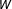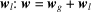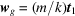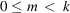International
Tables for
Crystallography
Volume A
Space-group symmetry
Edited by M. I. Aroyo

International Tables for Crystallography (2016). Vol. A, ch. 3.3, pp. 779-780

## Section 3.3.2. Space-group symbols

H. Burzlaffa and H. Zimmermannb*

aUniversität Erlangen–Nürnberg, Robert-Koch-Strasse 4a, D-91080 Uttenreuth, Germany, and bInstitut für Angewandte Physik, Lehrstuhl für Kristallographie und Strukturphysik, Universität Erlangen–Nürnberg, Bismarckstrasse 10, D-91054 Erlangen, Germany
Correspondence e-mail:  helmuth.zimmermann@krist.uni-erlangen.de

### 3.3.2. Space-group symbols

| top | pdf |

#### 3.3.2.1. Introduction

| top | pdf |

Each space group is related to a crystallographic point group. Space-group symbols, therefore, can be obtained by a modification of point-group symbols. The simplest modification which merely gives an enumeration of the space-group types (cf. Section 1.3.4.1) has been used by Schoenflies. The Shubnikov and Hermann–Mauguin symbols, however, reveal the glide or screw components of the symmetry operations and are designed in such a way that the nature of the symmetry elements and their relative locations can be deduced from the symbol. [A detailed discussion and listings of computer-adapted space-group symbols implemented in crystallographic software, such as the so-called Hall symbols (Hall, 1981a,b) or explicit symbols (Shmueli, 1984), can be found in Chapter 1.4of International Tables for Crystallography, Volume B (2008).]

#### 3.3.2.2. Schoenflies symbols

| top | pdf |

Space groups related to one point group are distinguished by adding a numerical superscript to the point-group symbol. Thus, the space groups related to the point groupare called,,.

#### 3.3.2.3. The role of translation parts in the Shubnikov and Hermann–Mauguin symbols

| top | pdf |

A crystallographic symmetry operation(cf. Chapter 1.2) is described by a pair of matricesW is called the rotation part, w describes the translation part and determines the translation vector w of the operation. The translation part w can be decomposed into a glide/screw partand a location part; here,determines the location of the corresponding symmetry element with respect to the origin. The glide/screw partmay be derived by projecting w on the space invariant under W, i.e. for rotations and reflections w is projected on the corresponding rotation axis or mirror plane. With matrix notation,is determined byand, where k is the order of W, the integers m are restricted byand t1 is the shortest lattice vector in the direction of t (for details, cf. Sections 1.2.2.4and 1.5.4.1). Space groups contain sets of screw and rotation axes or glide and mirror planes. A screw rotation is symbolized by km. The Shubnikov notation and the international notation use the same symbols for screw rotations. The symbols for glide reflections in both notations are listed in Table 3.3.2.1.

 Table 3.3.2.1| top | pdf | Symbols of glide planes in the Shubnikov and Hermann–Mauguin space-group symbols
Glide plane perpendicular toGlide vectorShubnikov symbolHermann–Mauguin symbol
b or ca
a or cb
a or b or abc
cn
an
bn
abn
cd
ad
bd
abd
a + bd

If the point-group symbol contains only one generator, the related space group is described completely by the Bravais lattice and a symbol corresponding to that of the point group in which rotations and reflections are replaced by screw rotations or glide reflections, if necessary. If, however, two or more operations generate the point group, it is necessary to have information on the mutual orientations and locations of the corresponding space-group symmetry elements, i.e. information on the location components. This is described in the following sections.

#### 3.3.2.4. Shubnikov symbols

| top | pdf |

For the description of the mutual orientation of symmetry elements, the same symbols as for point groups are applied. In space groups, however, the symmetry elements need not intersect. In this case, the orientational symbols · (dot), : (colon), / (slash) are modified to. The space-group symbol starts with a description of the lattice defined by the basis a, b, c. For centred cells, the vectors to the centring points are given first. The same letters are used for basis vectors related by symmetry. The relative orientations of the vectors are denoted by the orientational symbols introduced above. The description of the lattice given in parentheses is followed by symbols of the generating elements of the related point group. If necessary, the symbols of the symmetry operations are modified to indicate their glide/screw parts. The first generator is separated from the lattice description by an orientation symbol. If this generator represents a mirror or glide plane, the dot connects the plane with the last two vectors whereas the colon refers only to the last vector. If the generator represents a rotation or a rotoreflection, the colon orients the related axis perpendicular to the plane given by the last two vectors whereas the dot refers only to the last vector. Two generators are separated by the symbols mentioned above to denote their relative orientations and sites. To make this description unique for space groups related to point groupwith Bravais lattices cP and cF, it is necessary to use three generators instead of two:. For the sake of unification, this kind of description is extended to the remaining two space groups having Bravais lattice cI.

#### Example: Shubnikov symbol for the space group with Schoenflies symbolThe Bravais lattice is oI (orthorhombic, body-centred). Therefore, the symbol for the lattice basis isindicating that there is a centring vectorrelative to the conventional orthorhombic cell. This vector is oblique with respect to the basis vector c, which is orthogonal to the perpendicular pair a and b. The basis vectors have independent lengths and are thus indicated by different letters a, b and c in arbitrary sequence.

To complete the symbol of the space group, we consider the point group. Its Shubnikov symbol is. Parallel to the (a, b) plane, there is a glide planeand a mirror plane m. The latter is chosen as generator. From the screw axisand the rotation axis 2, both parallel to c, the latter is chosen as generator. The third generator can be a glide plane c perpendicular to b. Thus the Shubnikov symbol ofisThe list of all Shubnikov symbols is given in column 3 of Table 3.3.3.1.

#### 3.3.2.5. International short symbols

| top | pdf |

The international symbol of a space group consists of two parts, just like the Shubnikov symbol. The first part is a capital letter that describes the type of centring of the conventional cell. It is followed by a modified point-group symbol that refers to the lattice symmetry directions. Centring type and point-group symbol determine the Bravais type of the translation group (cf. Section 3.1.1) and thus the point group of the lattice and the appropriate lattice symmetry directions. To derive the short international symbol of a given space group, the short symbol of the related point group must be modified in such a way that not only the rotation parts of the generating operations but also their translation parts can be constructed. This can be done by the following procedure:

 (i) The glide/screw parts of generators and indicators are symbolized by applying the symbols for glide planes in Table 3.3.2.1and the appropriate rules for screw rotations. (ii) The generators are chosen in such a way that the related symmetry elements do intersect as far as possible. Exceptions may occur for space groups related to the pure rotation point groups 222, 422, 622, 23 and 432. In these cases, the axes of the generators may or may not intersect. (iii) Subgroups of lattice point groups may have lattice symmetry directions with which no symmetry elements are associated. Such symmetry directions are symbolized by 1'. This symbol can only be omitted if no ambiguity arises, e.g.is reduced to. P31m and P3m1, however, cannot be reduced. The use of the symbol 1' is discussed by Buerger (1967) and Donnay (1969, 1977).

#### Example

Again consider space group. The space group contains glide planes c and b perpendicular to the primary set, c and a normal to the secondary set of symmetry directions and m and n perpendicular to the tertiary set. To determine the short symbol, one generator must be chosen from each pair. The standardization rules (see the following section) lead to the symbol Ibam.

### References

International Tables for Crystallography (2008). Vol. B, Reciprocal Space. Edited by U. Shmueli, 3rd ed. Heidelberg: Springer.
Buerger, M. J. (1967). Some desirable modifications of the international symmetry symbols. Proc. Natl Acad. Sci. USA, 58, 1768–1773.
Donnay, J. D. H. (1969). Symbolism of rhombohedral space groups in Miller axes. Acta Cryst. A25, 715–716.
Donnay, J. D. H. (1977). The structural classification of crystal point symmetries. Acta Cryst. A33, 979–984.
Hall, S. R. (1981a). Space-group notation with an explicit origin. Acta Cryst. A37, 517–525.
Hall, S. R. (1981b). Space-group notation with an explicit origin; erratum. Acta Cryst. A37, 921.
Shmueli, U. (1984). Space-group algorithms. I. The space group and its symmetry elements. Acta Cryst. A40, 559–567.